# Meta-Analysis: Choose Your Model Wisely

## Why Do a Meta-Analysis?

Meta-Analyses are statistical techniques where we take the treatment effects from several studies and pool them to estimate the overall treatment effect.

This type of analysis is conducted so that we can get a precise estimate of the true treatment effect or estimate the mean of several true treatment effects and to notice how robust the effects are across studies. If the individual study treatment effects tend to vary substantially across the studies (true heterogeneity, separated from random error), then we want to explore the sources of this observed dispersion.

An excellent way to think of a meta-analysis is a large study. For example, if we have ten studies with 100 participants in each study, the inferences these studies can draw based on the small samples will be limited because of sampling error and we will get imprecise treatment effects and wide confidence intervals.

In theory, we can combine the results of all those ten studies to pretend that it’s like one giant study with 1,000 participants. Of course, there are a lot of caveats to this because things like quality control in the individual trials and sampling/measurement error will also play a significant role. More on that below.

In summary, we conduct a meta-analysis to get more precise treatment effects, to find how robust the effects are across a body of literature, and to explore sources of dispersion if they are indeed there.

When conducting a meta-analysis, there are two models that you can choose to go with, a common effects model or a random-effects model. These have entirely different definitions from other statistical scenarios, and I’ll try to explain them below.

First, remember that there are true effects (which we will never know, but what we’re trying to estimate) and there are observed effects, AKA the results we get from our studies. The circles are the true effects, what we’re trying to figure out or estimate. The boxes are the results we got. It’ll be a good idea to understand this or come back to this box so you understand the examples below, which are all taken from the bible of meta-analyses, Introduction to Meta-Analysis, by Michael Borenstein. I’d recommend it as a read, after you read this article of course.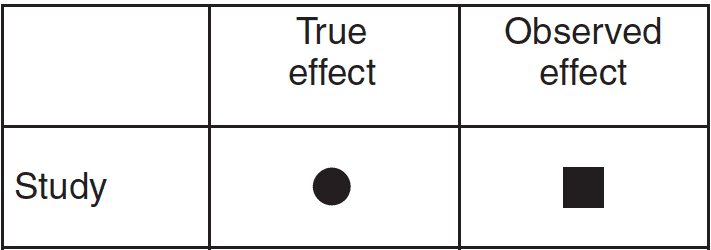## The Common-Effects (Fixed-Effects) Model

If a researcher chooses to go with a common effects model, he/she is going on the assumption that the studies being included are all incredibly similar. The participants were sampled from the same population; they used the same dosages for the intervention, same duration, and same protocols. Most things are meant to be similar, almost like a series of replication studies sampled from the same population.

So, we assume, that there is one real treatment effect (circles shown below) for the intervention when all these criteria (dosage, population, duration, protocol) are similar. AKA the true treatment effect is common for all the studies.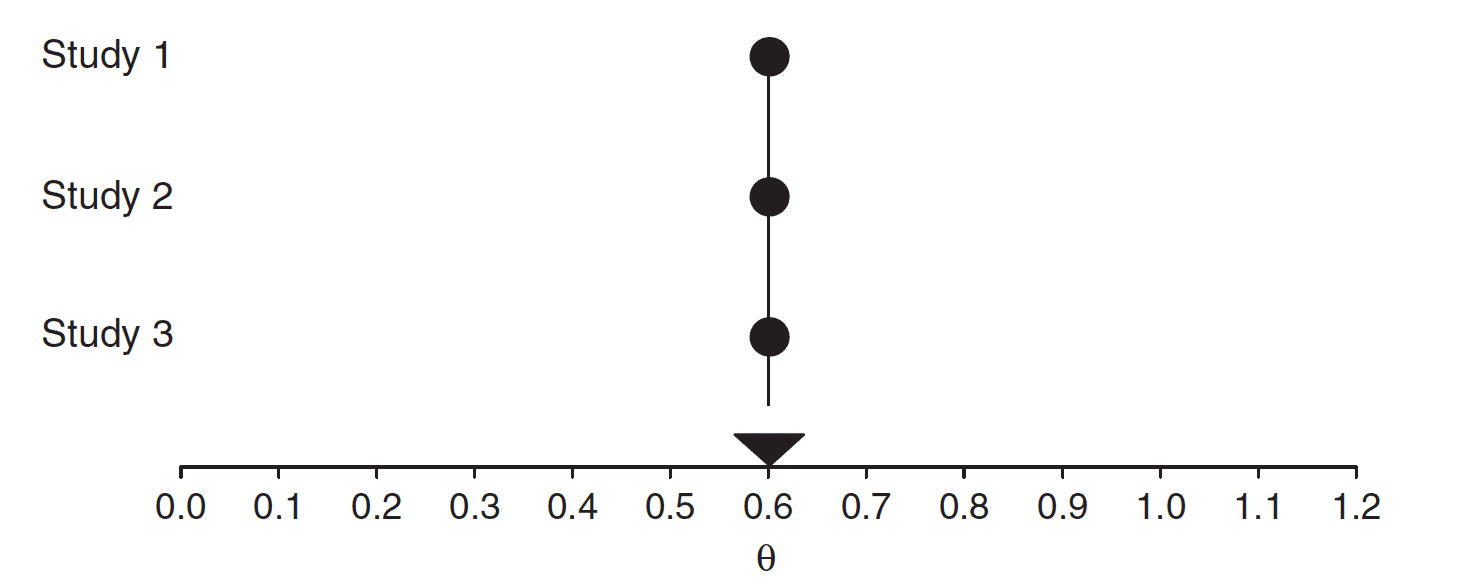But the observed treatment effects (the results we got, boxes) will differ from study to study, mainly due to sampling error.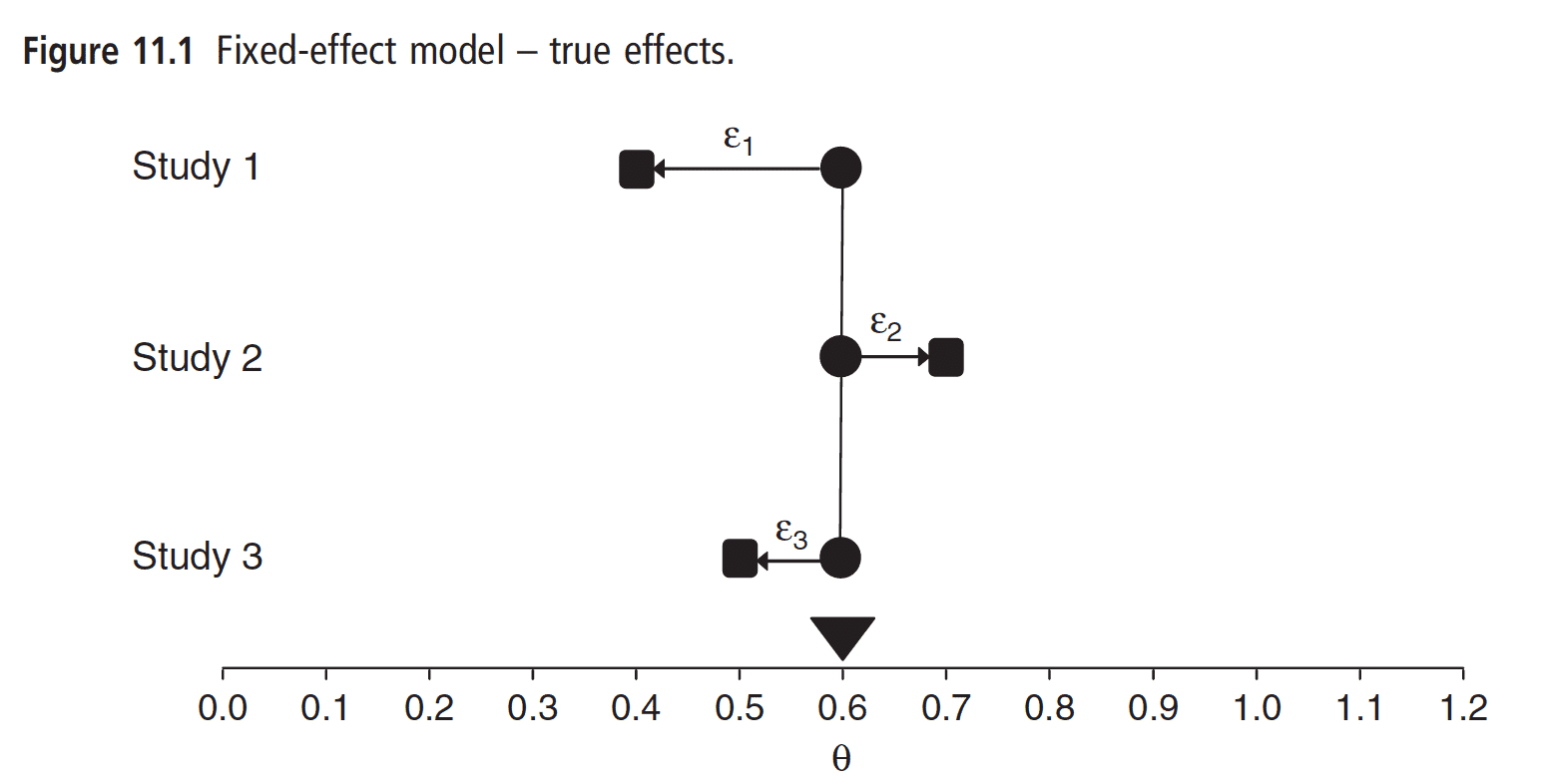Studies with smaller sample sizes have more sampling error, while larger ones have less. If our study had a sample size of infinity, the sampling error would practically be zero, and our observed treatment effect from this infinity study would be identical with the true treatment effect. Of course, this is usually not the case, and most people don’t have sample sizes of infinity.

So in a common effects model, we pool the results from several studies that are incredibly similar, with the assumption that smaller studies (which have more sampling error than larger studies) are less reliable than the larger studies. In this model, we assign more weight to the larger studies, which contribute more to the pooled effect, because they have less sampling error, and their observed treatment effects are likely to be closer to the true treatment effect. This can be seen below where the treatment effect from small studies have smaller boxes (smaller weights), and the larger studies have huge boxes (large weights).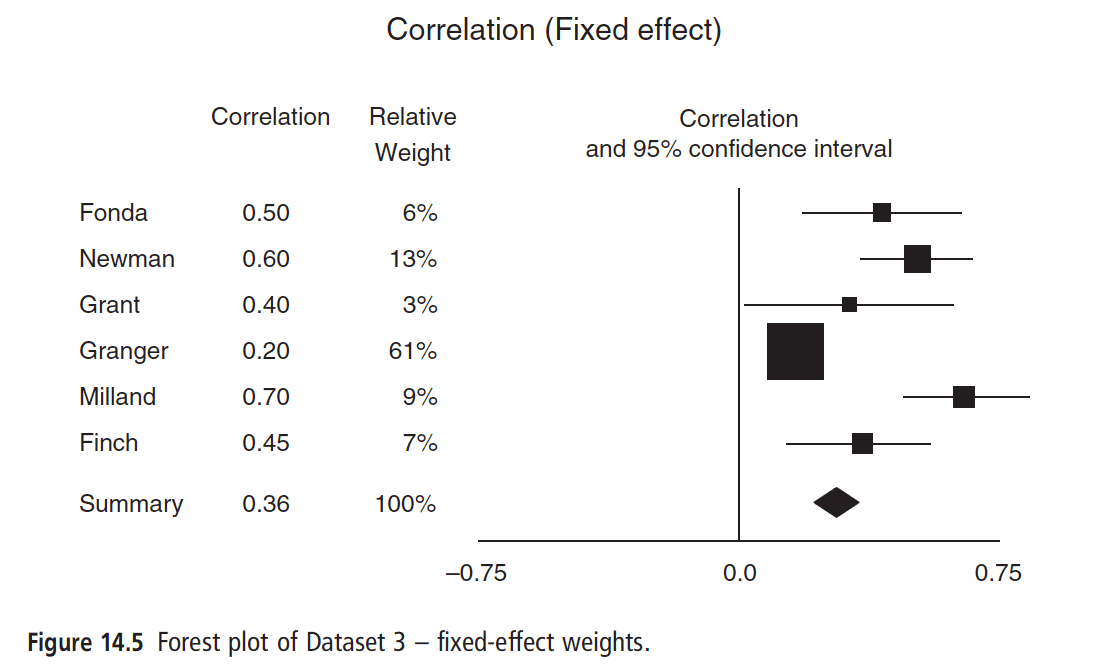So, small studies, with more extreme values in the observed treatment effects, will be less likely (hah!) to influence the overall treatment effect, which is trying to estimate what the TRUE treatment effect is.

Summary: Common effects models assume there is one true treatment effect and that all the studies included in the analysis are incredibly similar. Because the observed treatment effects from the individual studies will differ mainly due to sampling error, we put less weight on the smaller studies which are impacted more by sampling error, and we put more weight on the larger studies, which are less influenced by the random aspect of the sampling error.

## The Random-Effects Model

In a random-effects model, we are trying to estimate the mean treatment effect, from a distribution of observed treatment effects.

Let me unpack this. In a common effects meta-analysis, you assume all the studies are incredibly similar, and there is likely one TRUE treatment effect, and the only reason the studies differ in results is because of sampling error (hypothetically).

In a random-effects model, you are assuming that the studies are not very similar and that because things like dosages, durations, protocols, and interventions will differ, there will be several true treatment effects (see below) and your goal is to estimate the average of the treatment effects, which are normally distributed. This is more likely to represent real-world scenarios because there are rarely situations where a lot of the conditions between studies are highly similar.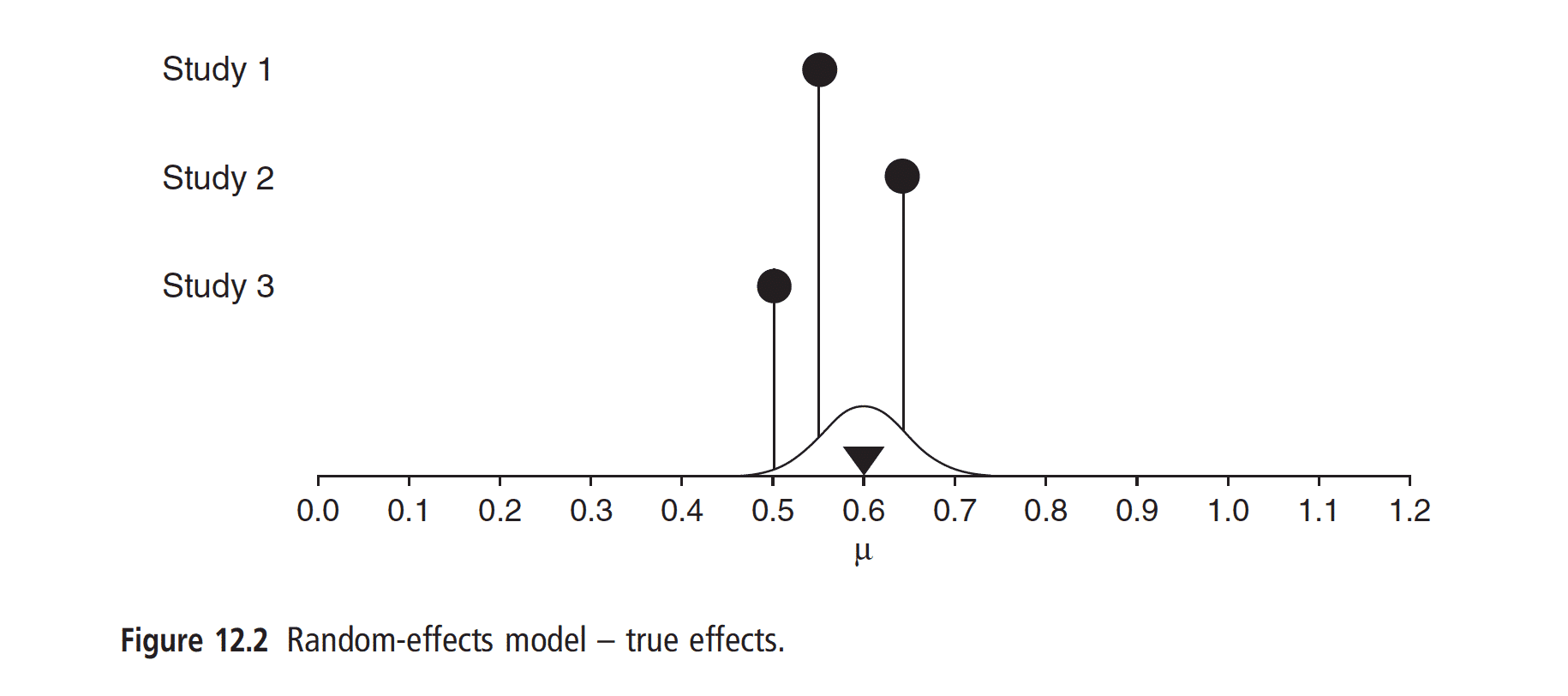Because each study provides a unique set of information, you do not merely assume that studies with smaller sample sizes are only influenced by sampling error and thus, less reliable than larger studies. No, now, you take into account that all of the studies likely differ, and provide a unique set of new information and that each study is individual, with its true treatment effect. And remember, we don’t have the true treatment effects (black circles) of the individual studies, we only have the observed effects (the results we got AKA the black boxes), which are influenced by sampling error amongst other things. See below.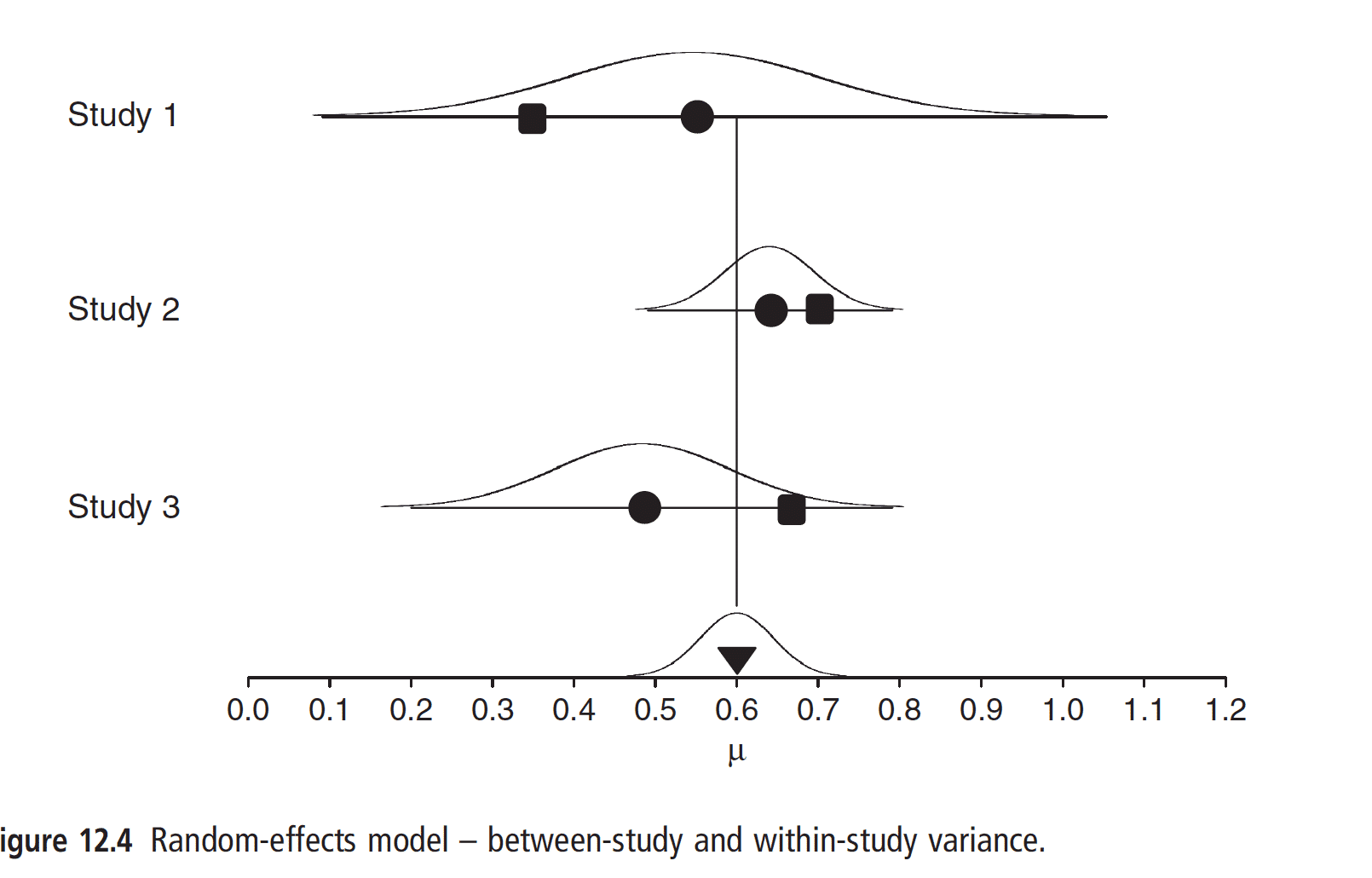So, you use a formula where the weights are more equally balanced amongst studies, even the small ones. Larger studies will still have more weight, but less so. Your goal now is not to discover the true one treatment effect, but instead, consider that there are several true treatment effects and you are trying to estimate the mean of these true treatment effects.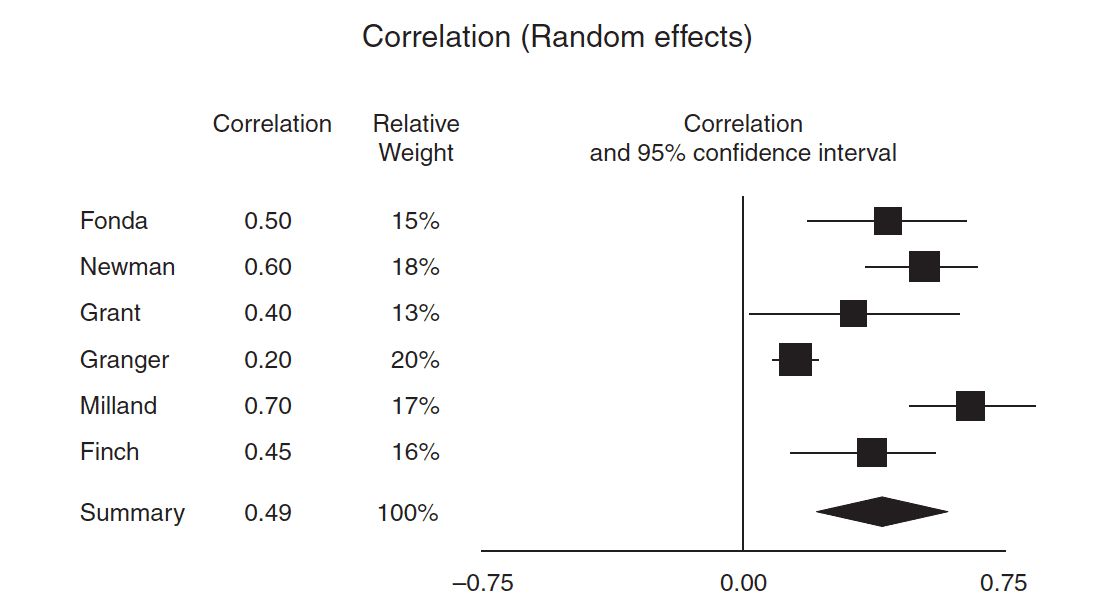Summary: In a random-effects model, you assume that the studies being included in your analysis differ in several aspects such as duration, dosage, population, protocols. Therefore you treat every study as being a new source of information, and you cannot merely assign larger studies more weight in your calculations. You use a different formula where the study weights are now far more balanced because you are trying to estimate the MEAN of the true treatment effects, which will differ from study to study.

## The Importance of Choosing the Right Model

So, why even use different models? Do they lead to different results? Yes, they do. Common effects models will often give you more precision and smaller intervals, again, because the formula you use is going off the assumption that the studies are incredibly similar. So, your interval will be narrow. In a random-effects model, because there are studies that are different, that each present new information, your intervals will be a lot wider, thus making you more uncertain. Here are some examples.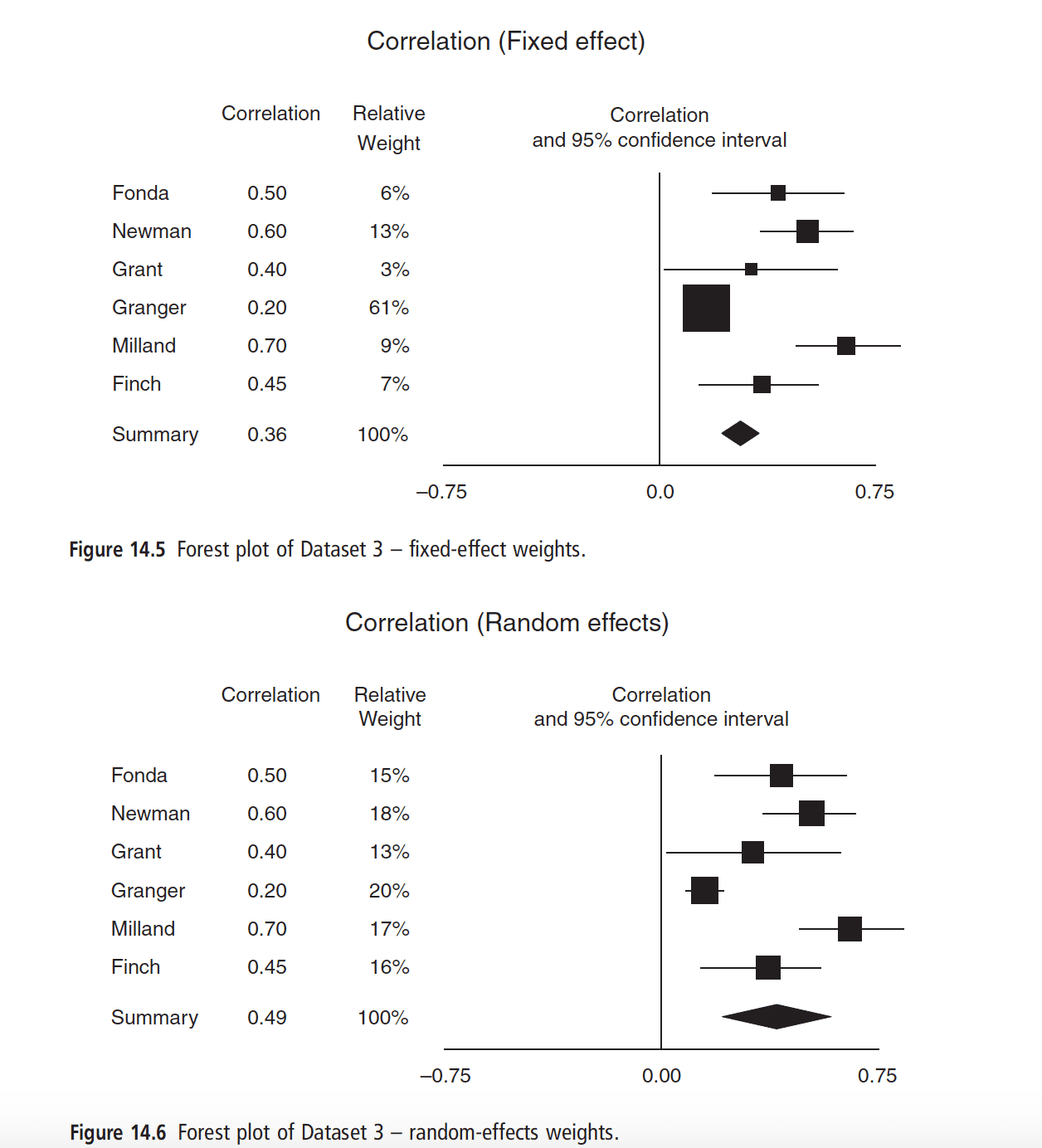Looked at the pooled treatment effects (the diamonds). Notice how much smaller and precise the common effects model one is. Now, look at random-effects model one. Way less precision!

Another example using dichotomous outcomes.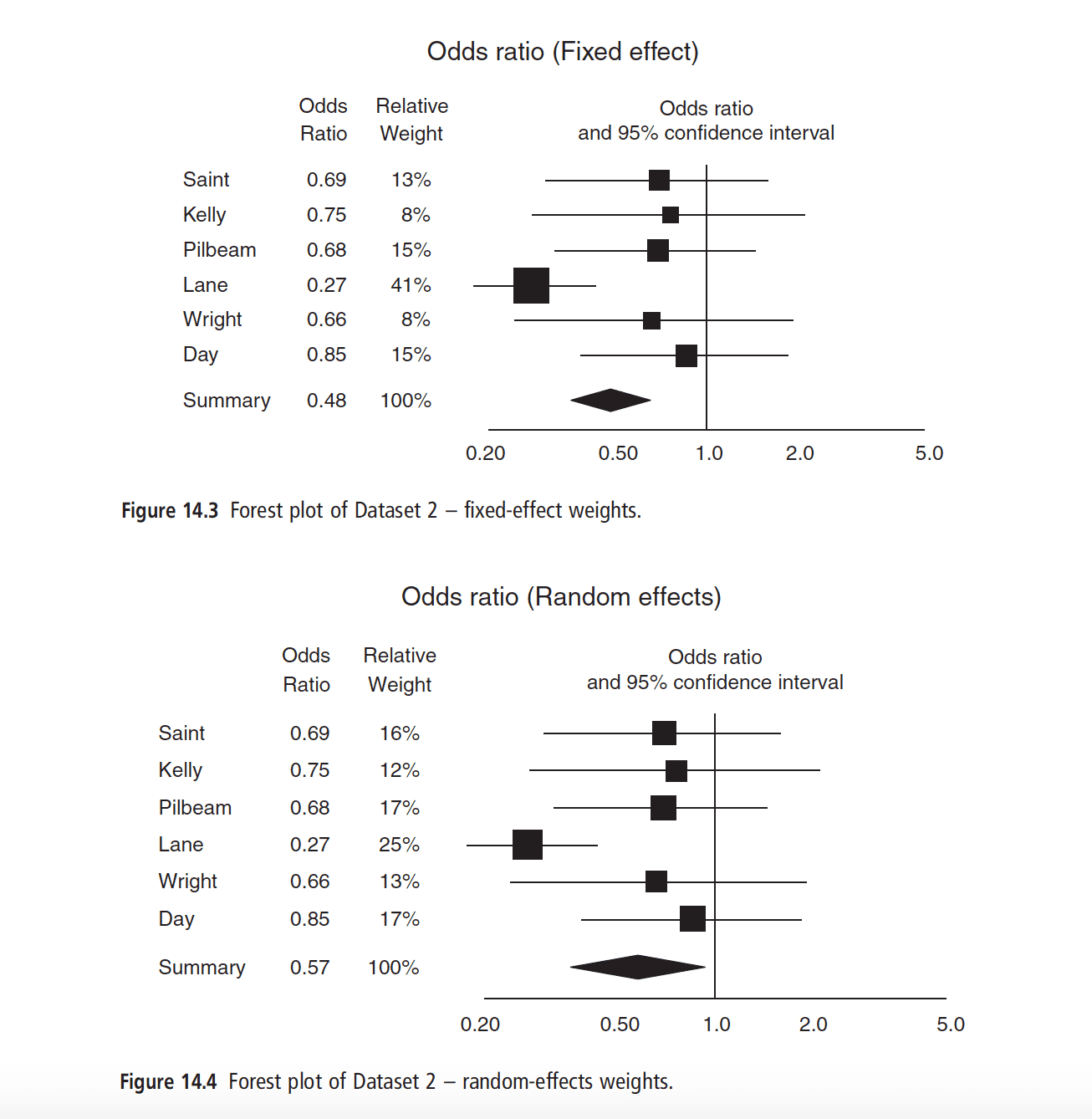## What Not to Do

The biggest mistake that researchers will do is try to figure out whether the studies they’re looking at are similar based on a test of homogeneity. If the test is significant, they assume that their studies are not similar, and they will switch to a random-effects model, but if the result is not significant, then they will stick with a common effects model.

This is problematic because the test of homogeneity often suffers from low power. Therefore it is more likely to lead to people going with common effects models when there is heterogeneity. The other problem is that choosing a model should not be based on tests of significance but rather through an understanding of the designs and effects of the studies being included.

## Prior Assumptions

If you know that the studies being included in your meta-analysis are very similar, have very similar protocols and similar effects, and you plan on extrapolating the results only to that population, go with a common effects model, where you put more weight on larger studies.

If you know that the studies being included in your meta-analysis are NOT too similar, have very different protocols, samples that differ by age, sampled from different populations, different intervention dosages, and durations, treat each study as a new source of information and assign more balanced weights.

And that is how you should choose your model. Not based on tests of significance, but on prior information.

• Cite this blog post